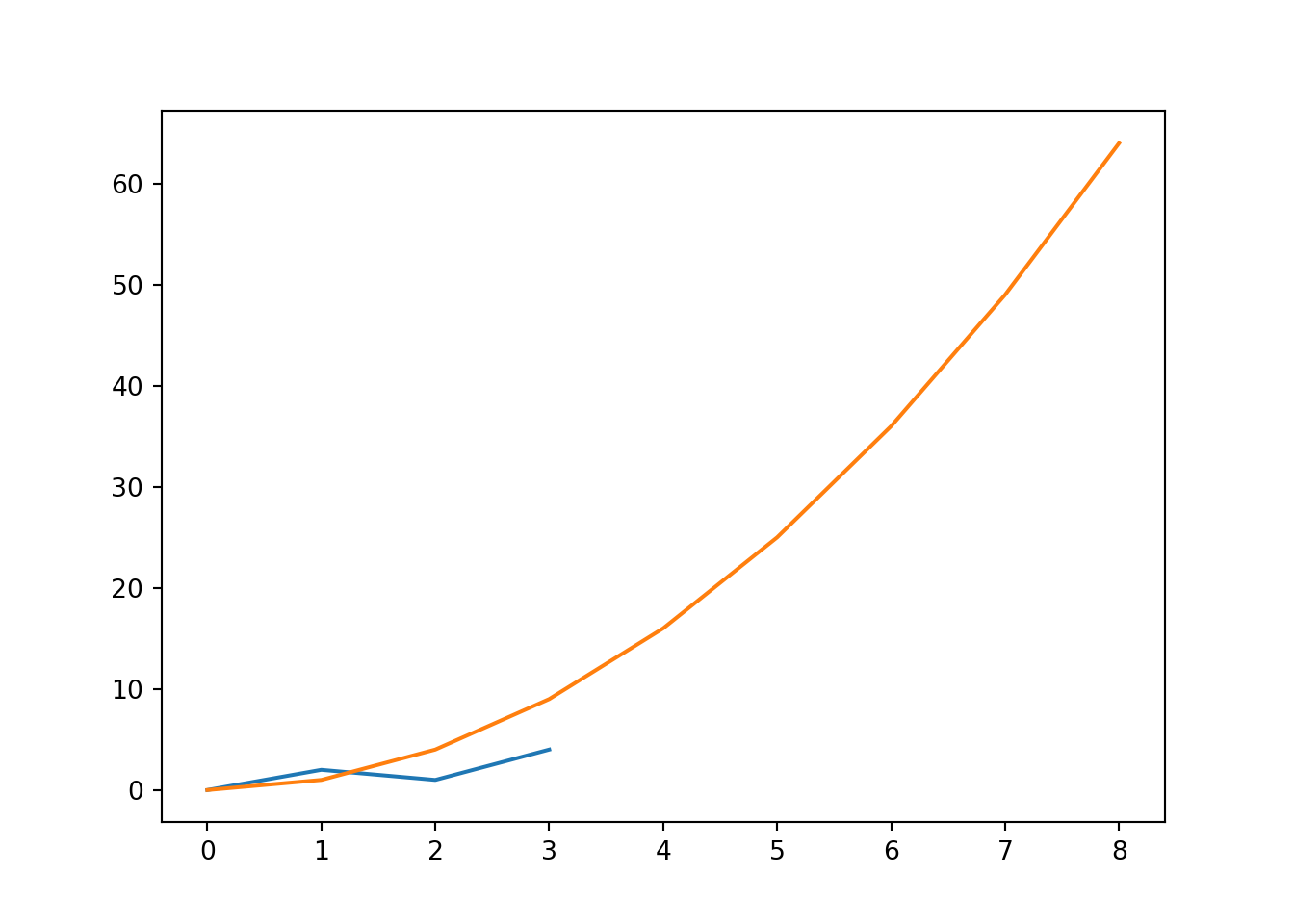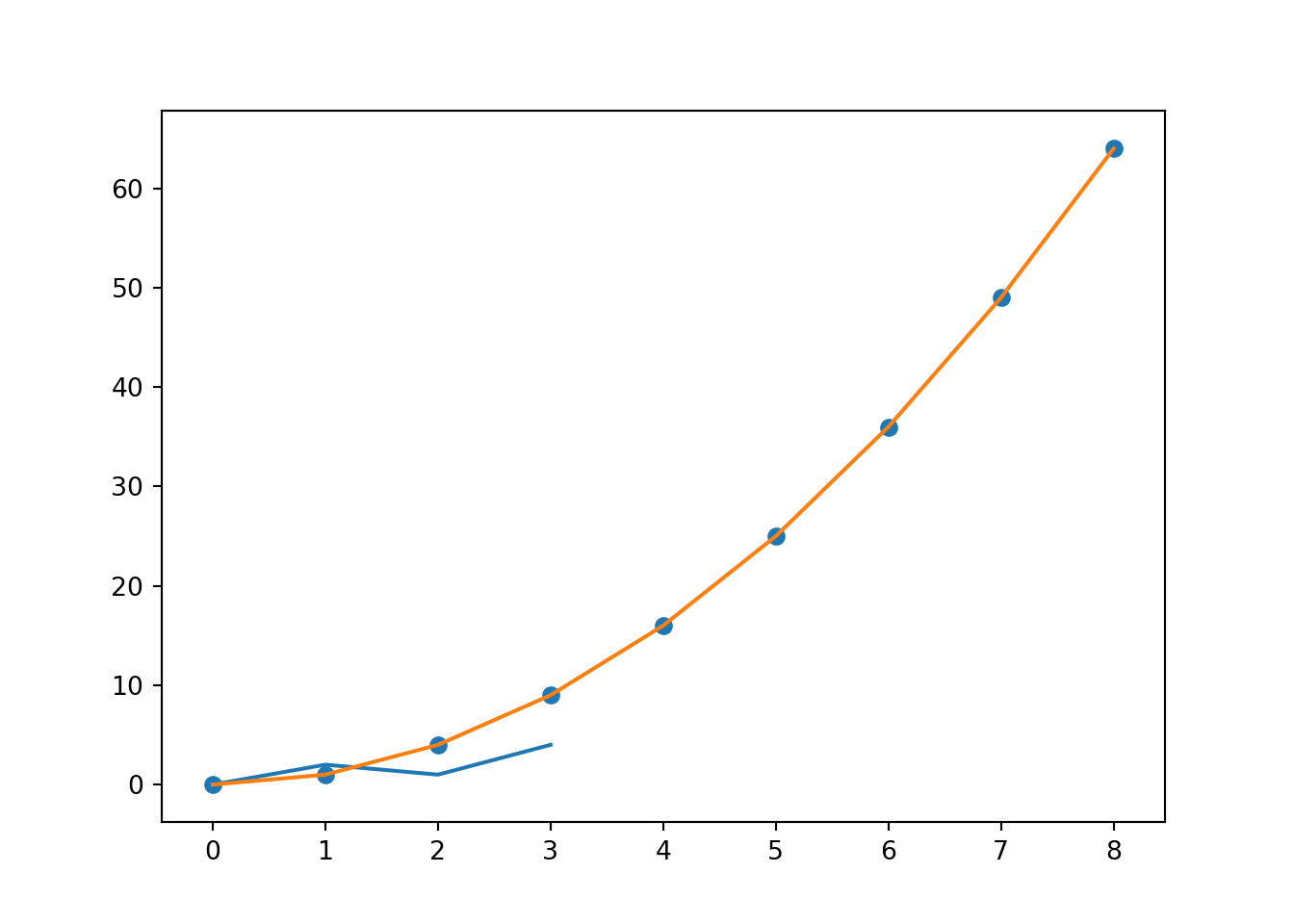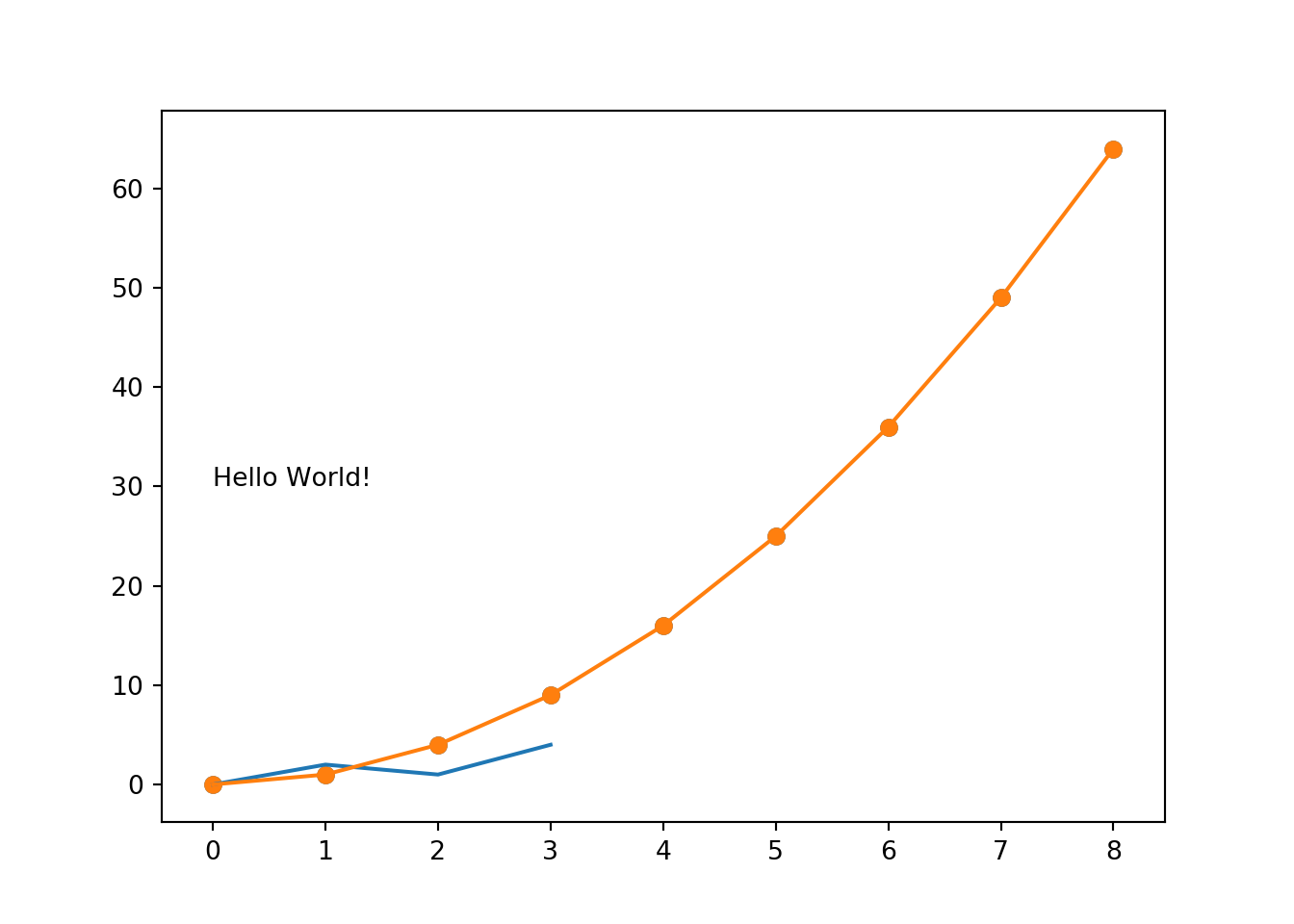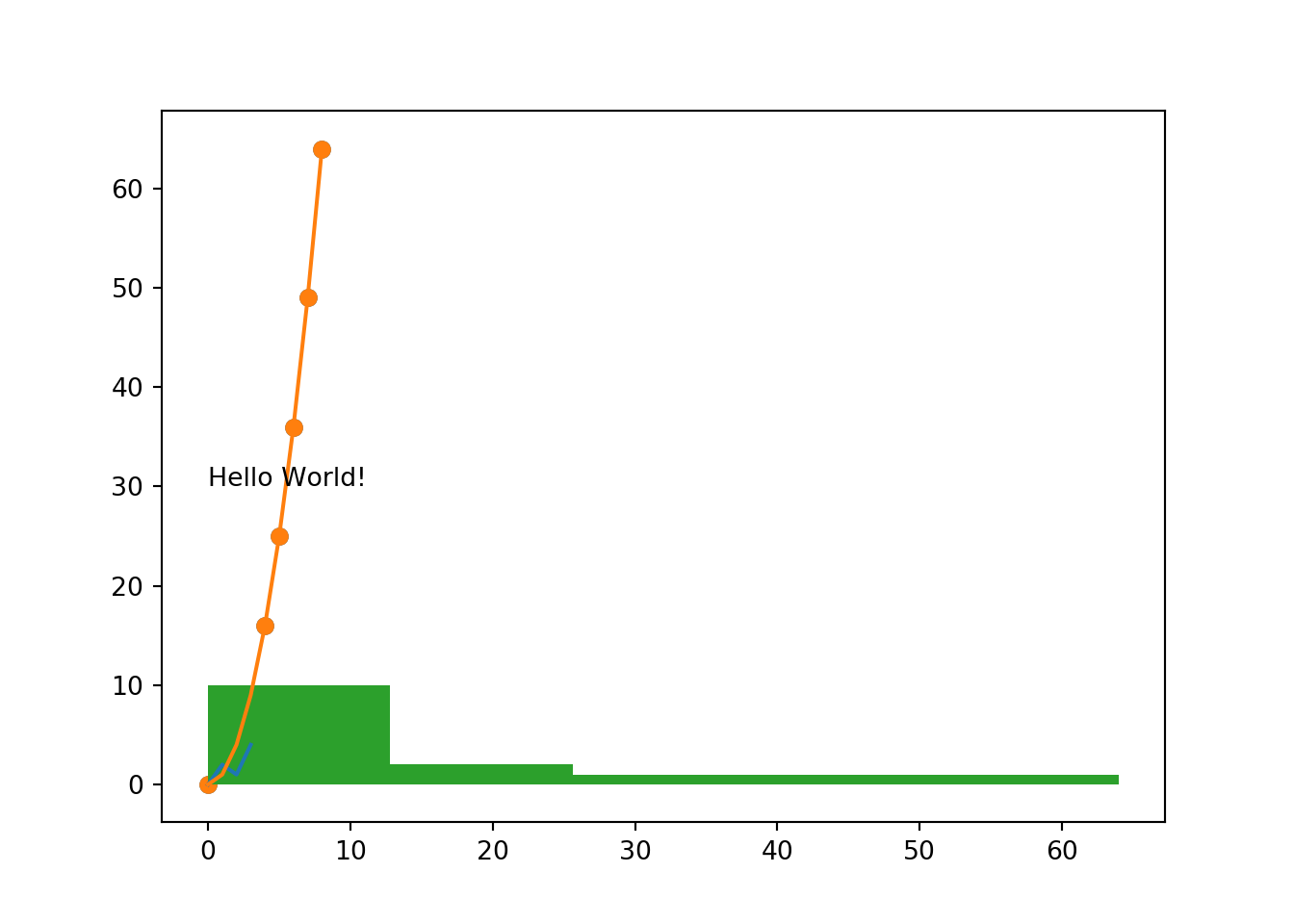# 8 Visualisation

Visualisation in Python is a bit of a mixed bag, with techniques ranging all the way from “just as bad as base R” to “could one day get close to ggplot2”.

## 8.1 Matplotlib

matplotlib has a sub-package called pyplot, and you will conventionally import this package as plt.

``import matplotlib.pyplot as plt``

When building plots with Matplotlib, you need to explicitly call `plt.show()` to print the plot to the console. If you want to clear the plot and start again you can use `plt.clf()`.

## 8.2 Line Plot

For a simple line plot, you can use `plt.plot(x, y)` where `x` and `y` are the arrays/lists corresponding to the data points for the x and y axes.

``````import matplotlib.pyplot as plt
x_val = [0,1,2,3,4,5,6,7,8]
y_val = [0,1,4,9,16,25,36,49,64]
plt.plot(x_val, y_val)
plt.show()``````## 8.3 Scatter Plot

Scatter plots are basically the same syntax as line plots, but with use the method `plt.scatter()`.

``````import matplotlib.pyplot as plt
x_val = [0,1,2,3,4,5,6,7,8]
y_val = [0,1,4,9,16,25,36,49,64]
plt.scatter(x_val, y_val)
plt.show()``````• `s` which gives the size of each point
• `c` which gives the colour of each point
• `alpha` which gives the opacity of each point

## 8.4 Text

You can add text to plots using the `plt.text()` function.

``````import matplotlib.pyplot as plt
x_val = [0,1,2,3,4,5,6,7,8]
y_val = [0,1,4,9,16,25,36,49,64]
plt.scatter(x_val, y_val)
plt.text(0, 30, "Hello World!")
plt.show()``````## 8.5 Histograms

Histograms are fairly straight-forward too: `plt.hist(x, bins)`.

``````import matplotlib.pyplot as plt
x = [0,1,2,3,4,5,6,7,8,9,16,25,36,49,64]
plt.hist(x, 5)
plt.show()``````## 8.6 Modifiers

### 8.6.1 Scales

• `plt.xscale('log')` converts the x scale to a logarithmic scale
• `plt.yscale('log')`
• `plt.grid(True)` displays the grid on the plot
• `plt.yticks([0,1,2,3], ["0", "1B", "2B", "3B"])` sets the Y axis ticks to the values given in the first argument, and sets the displayed strings on those ticks based on the second argument
• `plt.xticks([1000, 10000, 100000], ['1k', '10k', '100k'])`

### 8.6.2 Labels

• `plt.xlabel('Year')` sets the X axis label to “Year”
• `plt.ylabel('Population')`
• `plt.title('World Population Projections')` sets the title of the plot